# RD Sharma Solutions for Class 8 Maths Chapter 4 - Cubes and Cube Roots Exercise 4.5

Students can refer to RD Sharma Solutions Class 8 Maths which are designed by our expert team. Students can get easy and quick access to chapter-wise and exercise-wise solutions where students can practice a large number and different types of questions from the textbook. This would ensure they come out with flying colours in their exams. Exercise 4.5 of RD Sharma Class 8 Solutions for Chapter 4 Cubes and Cube Roots, we shall discuss problems based on finding cube roots using cube root tables. Download free pdf of RD Sharma Class 8 Maths from the links provided below.

## Download PDF of RD Sharma Solutions for Class 8 Maths Exercise 4.5 Chapter 4 Cubes and Cube Roots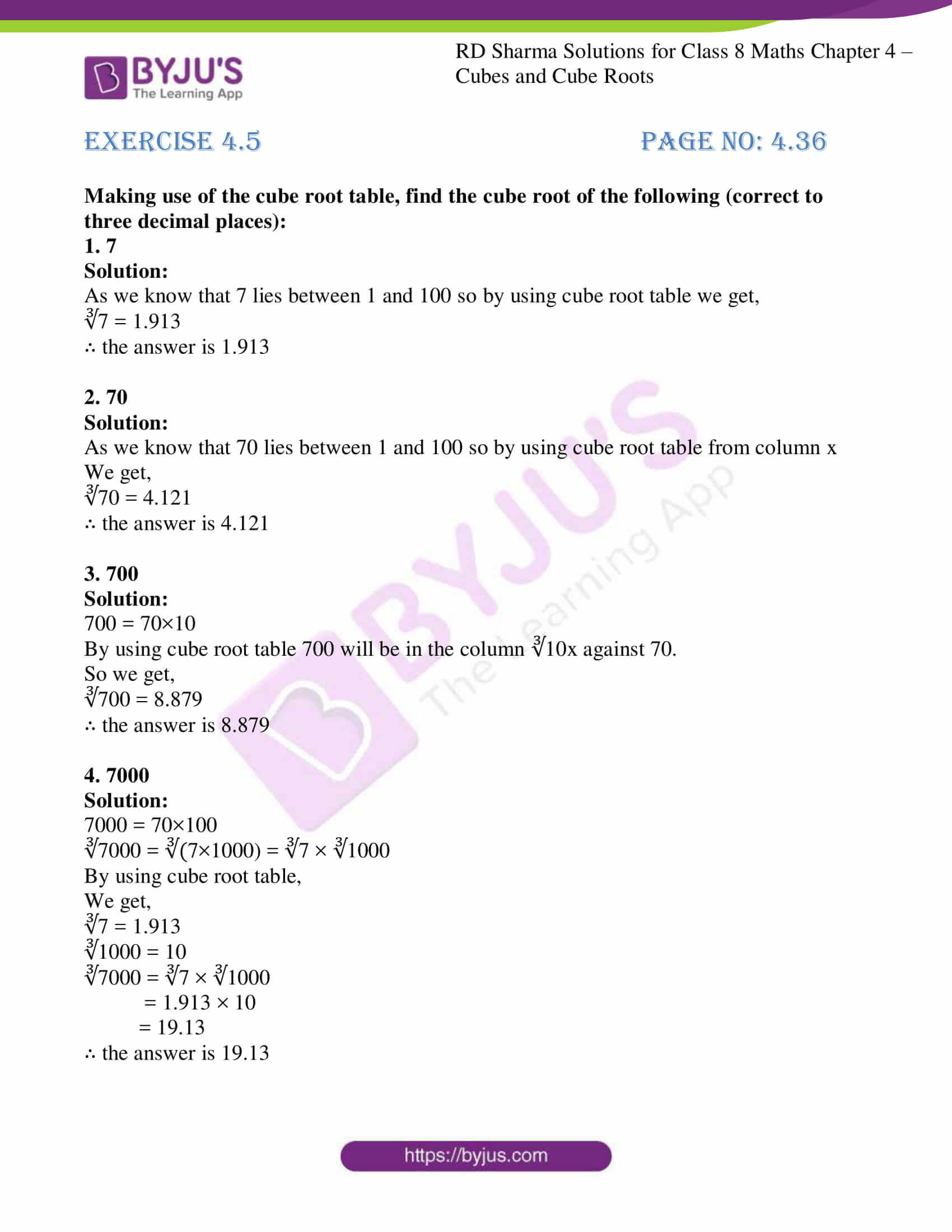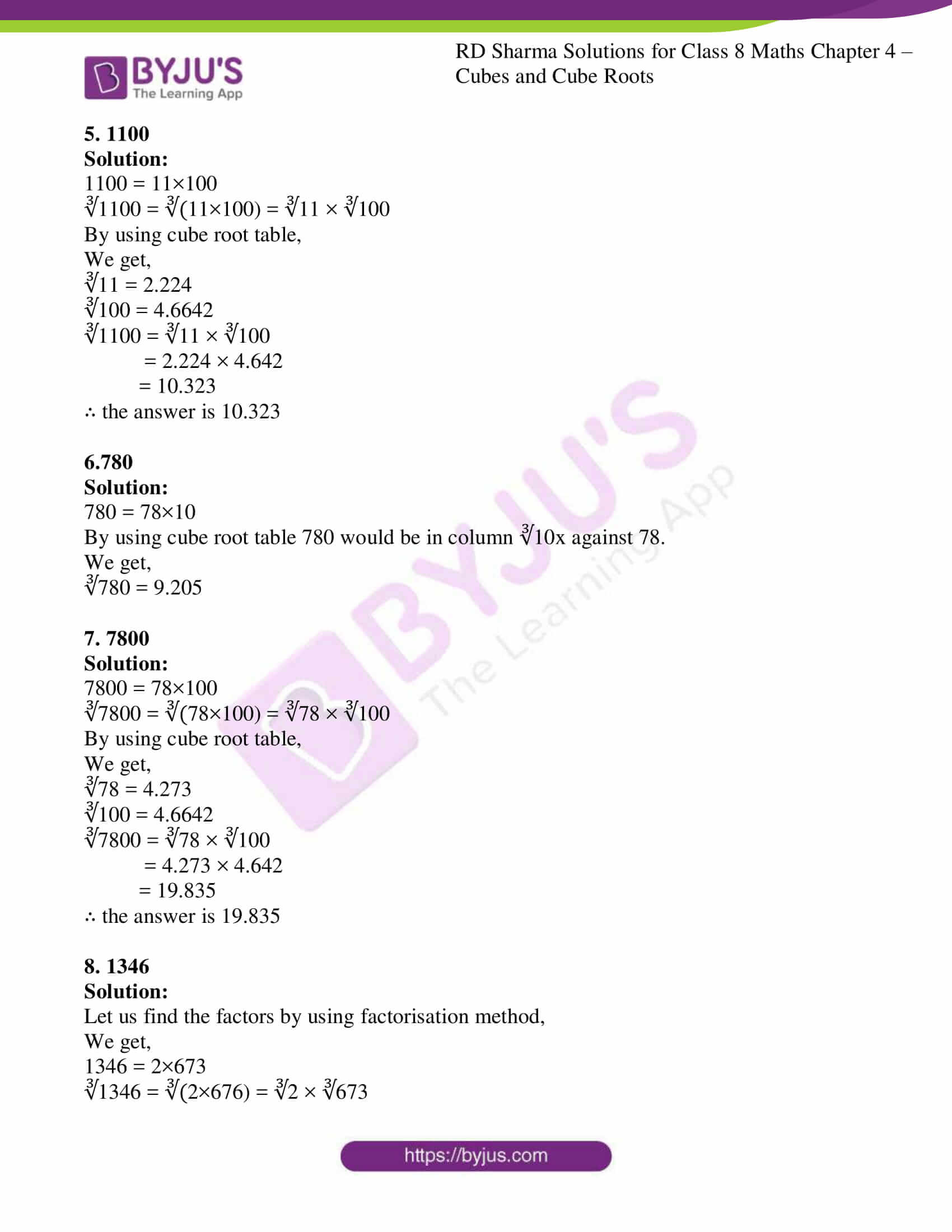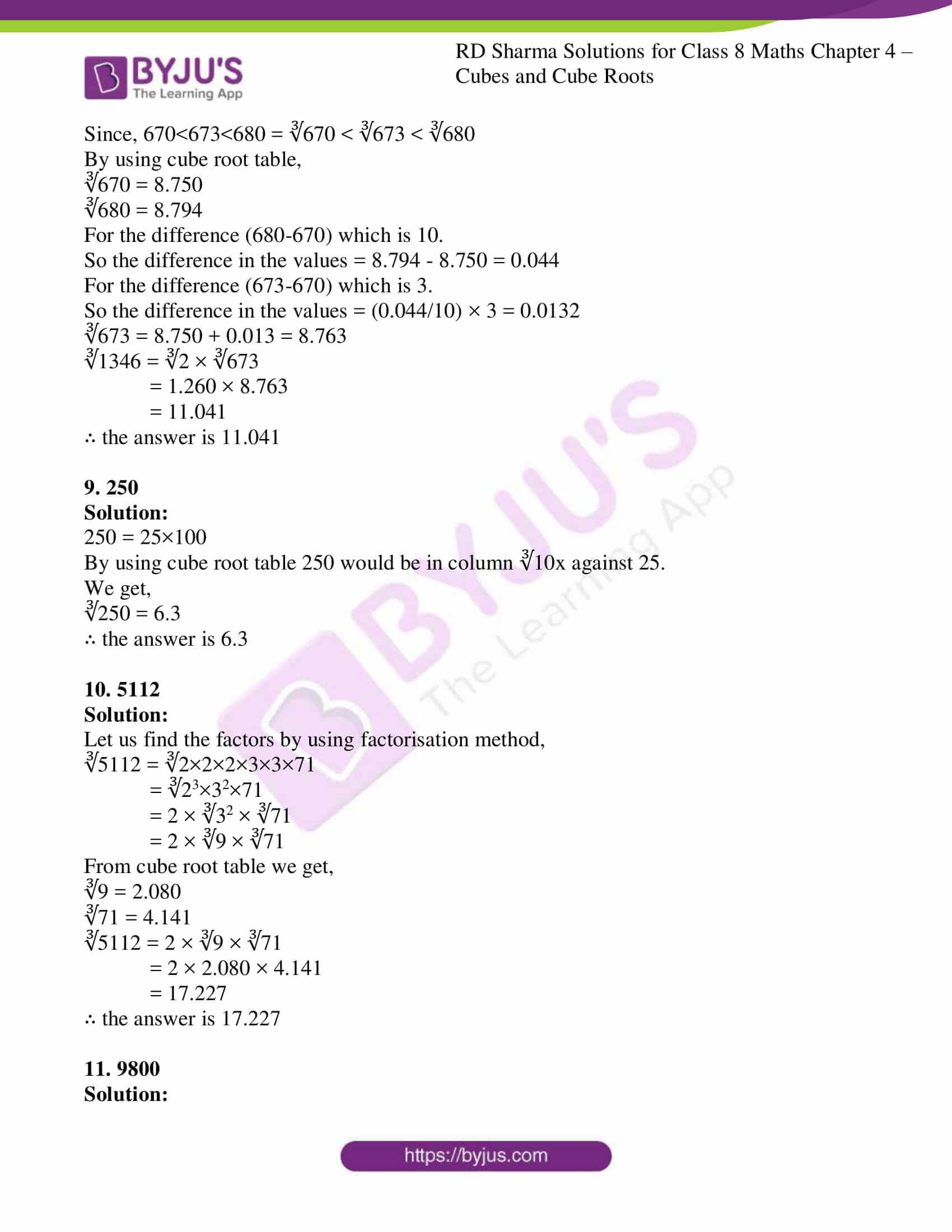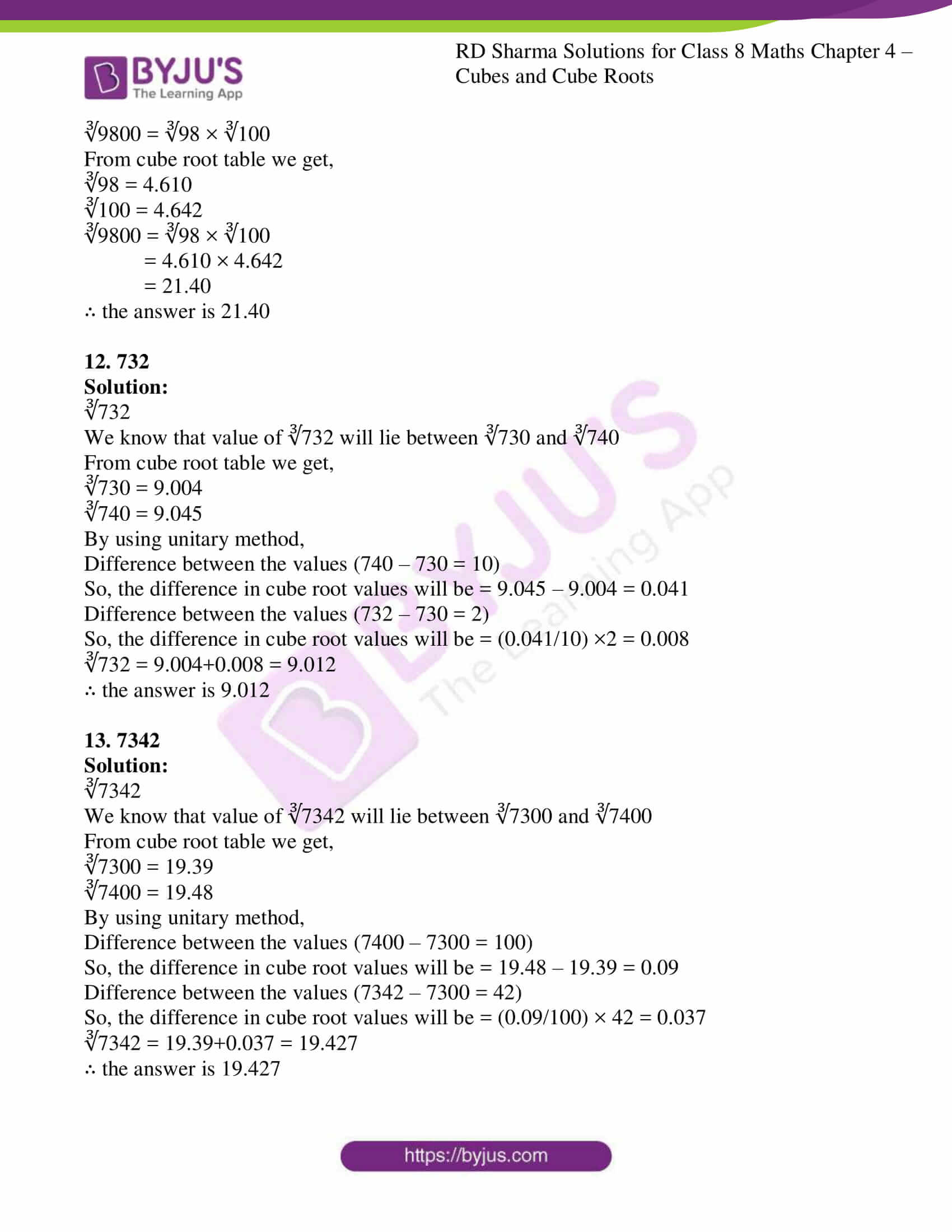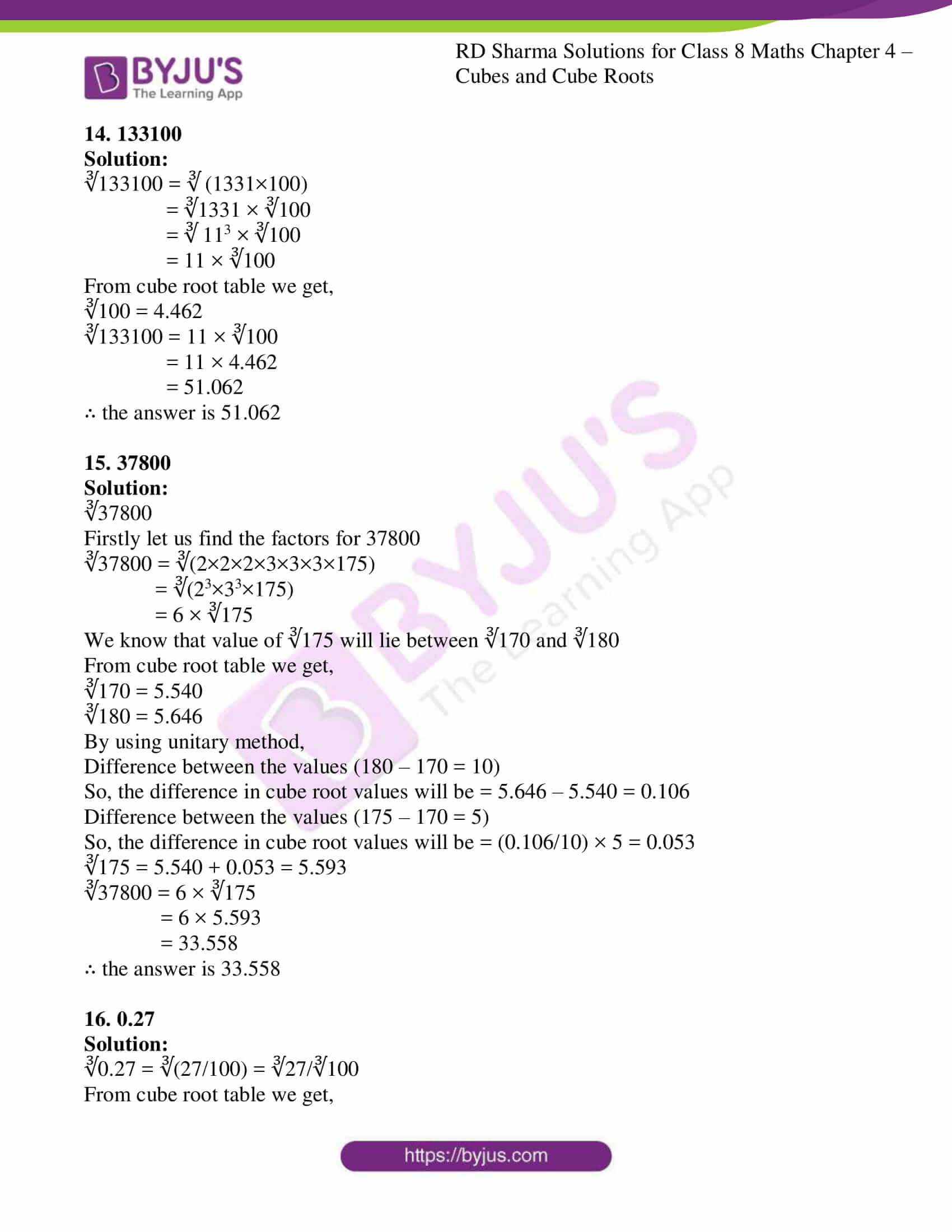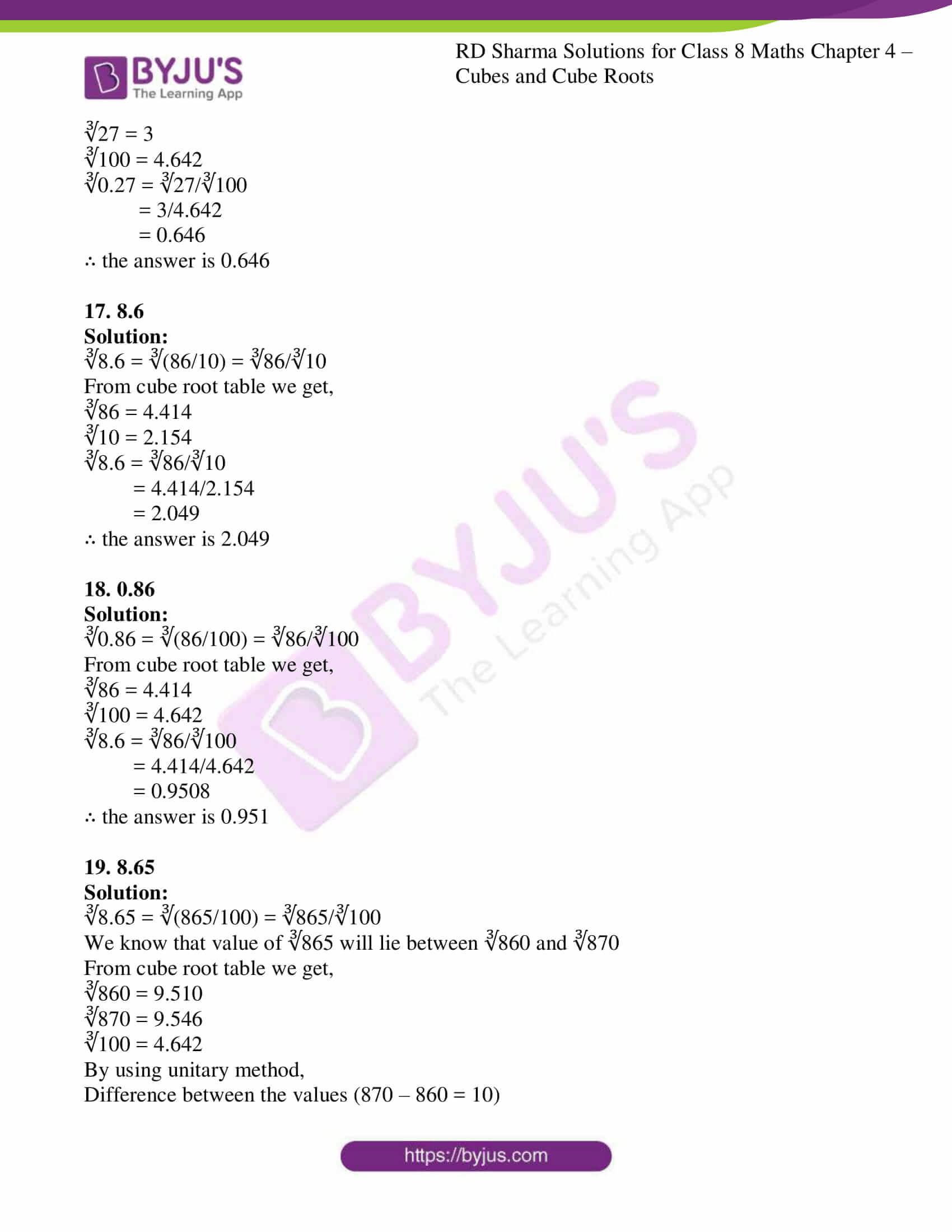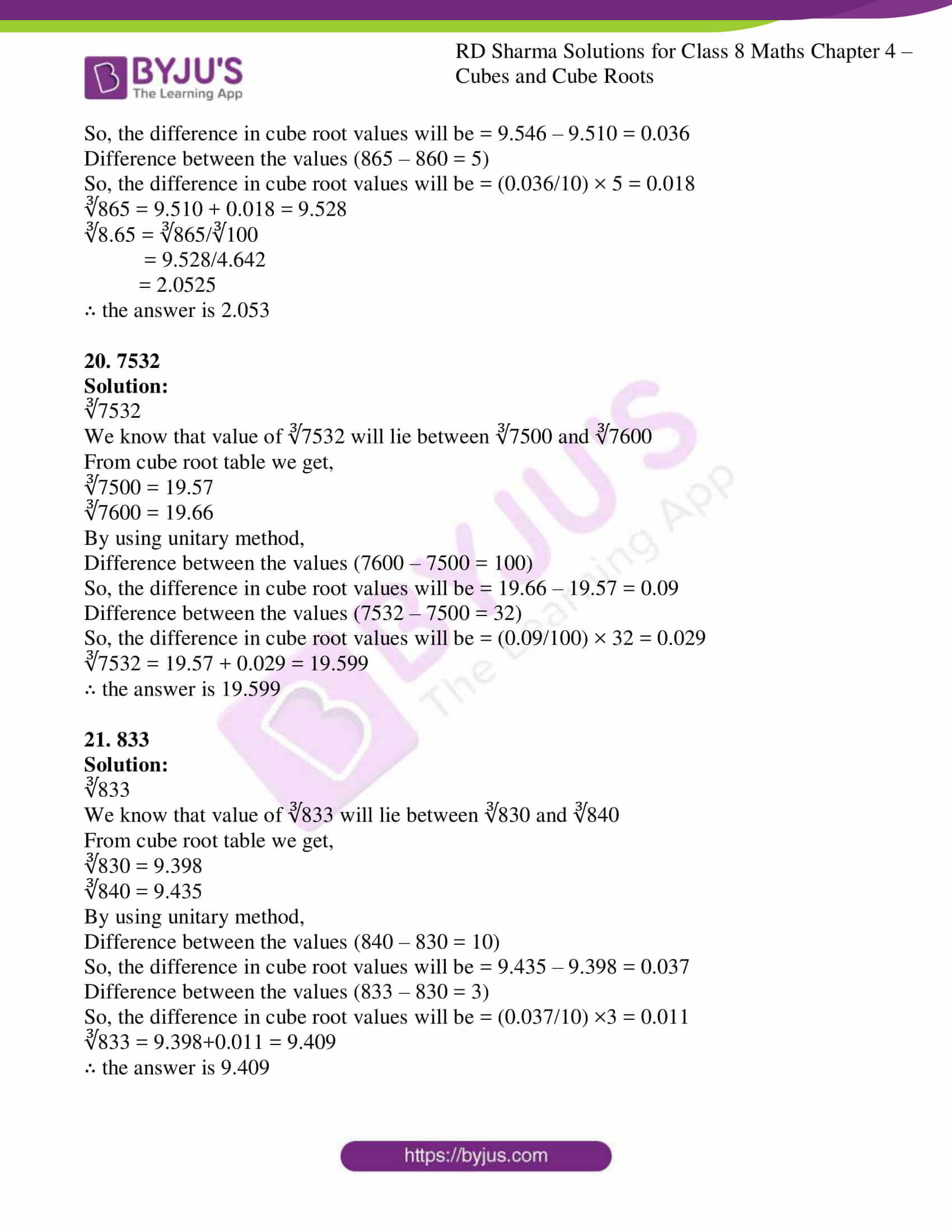### Access Answers to RD Sharma Solutions for Class 8 Maths Exercise 4.5 Chapter 4 Cubes and Cube Roots

Making use of the cube root table, find the cube root of the following (correct to three decimal places):

1. 7

Solution:

As we know that 7 lies between 1 and 100 so by using cube root table we get,

∛7 = 1.913

2. 70

Solution:

As we know that 70 lies between 1 and 100 so by using cube root table from column x

We get,

∛70 = 4.121

3. 700

Solution:

700 = 70×10

By using cube root table 700 will be in the column ∛10x against 70.

So we get,

∛700 = 8.879

4. 7000

Solution:

7000 = 70×100

∛7000 = ∛(7×1000) = ∛7 × ∛1000

By using cube root table,

We get,

∛7 = 1.913

∛1000 = 10

∛7000 = ∛7 × ∛1000

= 1.913 × 10

= 19.13

5. 1100

Solution:

1100 = 11×100

∛1100 = ∛(11×100) = ∛11 × ∛100

By using cube root table,

We get,

∛11 = 2.224

∛100 = 4.6642

∛1100 = ∛11 × ∛100

= 2.224 × 4.642

= 10.323

6.780

Solution:

780 = 78×10

By using cube root table 780 would be in column ∛10x against 78.

We get,

∛780 = 9.205

7. 7800

Solution:

7800 = 78×100

∛7800 = ∛(78×100) = ∛78 × ∛100

By using cube root table,

We get,

∛78 = 4.273

∛100 = 4.6642

∛7800 = ∛78 × ∛100

= 4.273 × 4.642

= 19.835

8. 1346

Solution:

Let us find the factors by using factorisation method,

We get,

1346 = 2×673

∛1346 = ∛(2×676) = ∛2 × ∛673

Since, 670<673<680 = ∛670 < ∛673 < ∛680

By using cube root table,

∛670 = 8.750

∛680 = 8.794

For the difference (680-670) which is 10.

So the difference in the values = 8.794 – 8.750 = 0.044

For the difference (673-670) which is 3.

So the difference in the values = (0.044/10) × 3 = 0.0132

∛673 = 8.750 + 0.013 = 8.763

∛1346 = ∛2 × ∛673

= 1.260 × 8.763

= 11.041

9. 250

Solution:

250 = 25×100

By using cube root table 250 would be in column ∛10x against 25.

We get,

∛250 = 6.3

10. 5112

Solution:

Let us find the factors by using factorisation method,

∛5112 = ∛2×2×2×3×3×71

= ∛23×32×71

= 2 × ∛32 × ∛71

= 2 × ∛9 × ∛71

From cube root table we get,

∛9 = 2.080

∛71 = 4.141

∛5112 = 2 × ∛9 × ∛71

= 2 × 2.080 × 4.141

= 17.227

11. 9800

Solution:

∛9800 = ∛98 × ∛100

From cube root table we get,

∛98 = 4.610

∛100 = 4.642

∛9800 = ∛98 × ∛100

= 4.610 × 4.642

= 21.40

12. 732

Solution:

∛732

We know that value of ∛732 will lie between ∛730 and ∛740

From cube root table we get,

∛730 = 9.004

∛740 = 9.045

By using unitary method,

Difference between the values (740 – 730 = 10)

So, the difference in cube root values will be = 9.045 – 9.004 = 0.041

Difference between the values (732 – 730 = 2)

So, the difference in cube root values will be = (0.041/10) ×2 = 0.008

∛732 = 9.004+0.008 = 9.012

13. 7342

Solution:

∛7342

We know that value of ∛7342 will lie between ∛7300 and ∛7400

From cube root table we get,

∛7300 = 19.39

∛7400 = 19.48

By using unitary method,

Difference between the values (7400 – 7300 = 100)

So, the difference in cube root values will be = 19.48 – 19.39 = 0.09

Difference between the values (7342 – 7300 = 42)

So, the difference in cube root values will be = (0.09/100) × 42 = 0.037

∛7342 = 19.39+0.037 = 19.427

14. 133100

Solution:

∛133100 = ∛ (1331×100)

= ∛1331 × ∛100

= ∛ 113 × ∛100

= 11 × ∛100

From cube root table we get,

∛100 = 4.462

∛133100 = 11 × ∛100

= 11 × 4.462

= 51.062

15. 37800

Solution:

∛37800

Firstly let us find the factors for 37800

∛37800 = ∛(2×2×2×3×3×3×175)

= ∛(23×33×175)

= 6 × ∛175

We know that value of ∛175 will lie between ∛170 and ∛180

From cube root table we get,

∛170 = 5.540

∛180 = 5.646

By using unitary method,

Difference between the values (180 – 170 = 10)

So, the difference in cube root values will be = 5.646 – 5.540 = 0.106

Difference between the values (175 – 170 = 5)

So, the difference in cube root values will be = (0.106/10) × 5 = 0.053

∛175 = 5.540 + 0.053 = 5.593

∛37800 = 6 × ∛175

= 6 × 5.593

= 33.558

16. 0.27

Solution:

∛0.27 = ∛(27/100) = ∛27/∛100

From cube root table we get,

∛27 = 3

∛100 = 4.642

∛0.27 = ∛27/∛100

= 3/4.642

= 0.646

17. 8.6

Solution:

∛8.6 = ∛(86/10) = ∛86/∛10

From cube root table we get,

∛86 = 4.414

∛10 = 2.154

∛8.6 = ∛86/∛10

= 4.414/2.154

= 2.049

18. 0.86

Solution:

∛0.86 = ∛(86/100) = ∛86/∛100

From cube root table we get,

∛86 = 4.414

∛100 = 4.642

∛8.6 = ∛86/∛100

= 4.414/4.642

= 0.9508

19. 8.65

Solution:

∛8.65 = ∛(865/100) = ∛865/∛100

We know that value of ∛865 will lie between ∛860 and ∛870

From cube root table we get,

∛860 = 9.510

∛870 = 9.546

∛100 = 4.642

By using unitary method,

Difference between the values (870 – 860 = 10)

So, the difference in cube root values will be = 9.546 – 9.510 = 0.036

Difference between the values (865 – 860 = 5)

So, the difference in cube root values will be = (0.036/10) × 5 = 0.018

∛865 = 9.510 + 0.018 = 9.528

∛8.65 = ∛865/∛100

= 9.528/4.642

= 2.0525

20. 7532

Solution:

∛7532

We know that value of ∛7532 will lie between ∛7500 and ∛7600

From cube root table we get,

∛7500 = 19.57

∛7600 = 19.66

By using unitary method,

Difference between the values (7600 – 7500 = 100)

So, the difference in cube root values will be = 19.66 – 19.57 = 0.09

Difference between the values (7532 – 7500 = 32)

So, the difference in cube root values will be = (0.09/100) × 32 = 0.029

∛7532 = 19.57 + 0.029 = 19.599

21. 833

Solution:

∛833

We know that value of ∛833 will lie between ∛830 and ∛840

From cube root table we get,

∛830 = 9.398

∛840 = 9.435

By using unitary method,

Difference between the values (840 – 830 = 10)

So, the difference in cube root values will be = 9.435 – 9.398 = 0.037

Difference between the values (833 – 830 = 3)

So, the difference in cube root values will be = (0.037/10) ×3 = 0.011

∛833 = 9.398+0.011 = 9.409

22. 34.2

Solution:

∛34.2 = ∛(342/10) = ∛342/∛10

We know that value of ∛342 will lie between ∛340 and ∛350

From cube root table we get,

∛340 = 6.980

∛350 = 7.047

∛10 = 2.154

By using unitary method,

Difference between the values (350 – 340 = 10)

So, the difference in cube root values will be = 7.047 – 6.980 = 0.067

Difference between the values (342 – 340 = 2)

So, the difference in cube root values will be = (0.067/10) × 2 = 0.013

∛342 = 6.980 + 0.013 = 6.993

∛34.2 = ∛342/∛10

= 6.993/2.154

= 3.246

23. What is the length of the side of a cube whose volume is 275 cm3. Make use of the table for the cube root.

Solution:

The given volume of the cube = 275cm3

Let us consider the side of the cube as ‘a’cm

a3 = 275

a = ∛275

We know that value of ∛275 will lie between ∛270 and ∛280

From cube root table we get,

∛270 = 6.463

∛280 = 6.542

By using unitary method,

Difference between the values (280 – 270 = 10)

So, the difference in cube root values will be = 6.542 – 6.463 = 0.079

Difference between the values (275 – 270 = 5)

So, the difference in cube root values will be = (0.079/10) × 5 = 0.0395

∛275 = 6.463 + 0.0395 = 6.5025